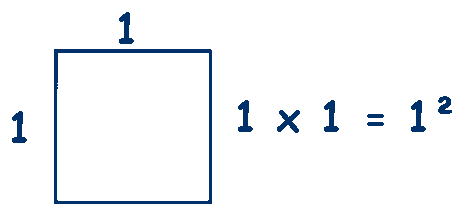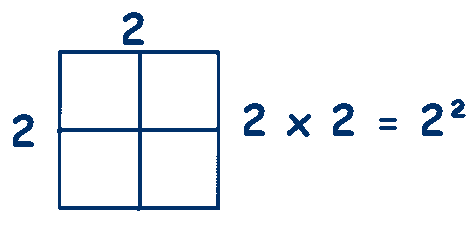# Square Numbers

## A Square Number is a number that is multiplied by itself.

### They are called square numbers because these numbers do form squares.The smallest square we can make with whole numbers (integers) is 1 unit by 1 unit

## 1 x 1 = 1² = 1

### so 1 is the first square number

The next square we can make with whole numbers (integers) is 2 by 2

## 2 x 2 = 2² = 4

### so 4 is the next square number

The next square we can make with whole numbers (integers) is 3 by 3

## 3 x 3 = 3² = 9

### so 9 is the next square number

I usually work through all the numbers up to 10, it doesn't take long after they get the idea and you end up with the first ten square numbers.

## 1, 4, 9, 16, 25, 36, 49, 64, 81 and 100

It is worth remembering these numbers as they will come up again and again.

Another useful idea is to highlight all the square numbers on a multiplication chart.
This Square Numbers worksheet from Mathsblog does just this.